# Implement Stack using Queues

Key takeaway: An excellent problem to visualize the use case of stack and queue operations.

### Let’s understand the problem

Write a program to implement a stack using the queues. The implemented stack should support standard operations like push(x), pop(), and top().

• void push(int x): insert element x to the top of the stack.
• int pop(): remove the element from the top of the stack and returns it.
• int top(): return the element on the top of the stack.

Problem Notes

• Stack is a last-in-first-out (LIFO) data structure, in which elements are added and removed from the top end. In contrast, the queue is a first-in-first-out (FIFO) data structure, in which elements are added from one end (back) and removed from the other end(front).
• We must use only standard queue operations like enqueue(x), dequeue(), front(), size(), and isEmpty().

### Discussed solution approaches

• Using two queues: O(n) pop operation and O(1) push operation
• Using two queues: O(1) pop operation and O(n) push operation
• Using a single queue: O(1) pop operation and O(n) push operation

### Using two queues: O(n) pop operation and O(1) push operation

We can get the basic solution idea by understanding the order of insertion and deletion from the stack and queue. In a stack, we insert and delete elements from one end only, but in a queue, we insert elements at the back and delete elements from the front. So for simulating a stack using the queue, we need to track the last inserted element and ensure that the last element must be the first element to be deleted. Think!

Suppose we use one queue to implement the stack, then we can perform a push operation by simply inserting every new element into the back of the queue. But if we try to perform the pop operation, then we need to remove the last inserted element in the queue i.e. element present at the back. But queue is a FIFO data structure, where we remove elements from the front of the queue. The critical question is: how do we remove the element at the back of the queue?

One idea would be: continuously remove elements from the queue till we reach the back element i.e. remove all the elements except the last element. Now there will be only one element left in the queue, which would be the top element of the stack. But before removing the last element, we need to store and maintain the order of the removed elements for future processing. So we need to use an additional queue to store the removed elements. So for performing the pop operation, we need to use two queues. Think!

Suppose we defined a class StackUsingQueue to implement stack using queues. Inside this class:

• We declare two queues Q1 and Q2, to simulate the stack operations.
• We declare a variable stackSize to track the current size of the stack.
• We define methods for stack operations: void push(int x), int pop() and int top().

Implementation of the push operation

We enqueue the new element to the first queue Q1. In other words, we add the new element x to the back of queue Q1, which will be the top element of the stack. After the insertion, we also increment the stackSize by 1.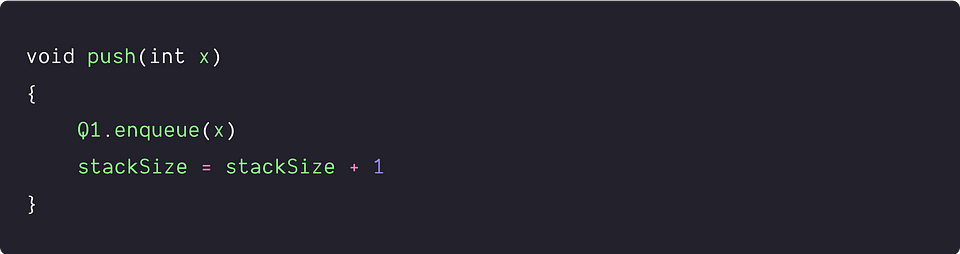• We are performing a constant number of operations i.e. only one enqueue operation. So time complexity = O(1)
• We are using constant extra space. So space complexity = O(1)

Implementation of the pop operation

As mentioned earlier, to perform the pop operation, our goal is to remove the last inserted element from Q1 using another queue (Q2). What should we do? Let's think!

Except for the last element, we move all elements of the first queue Q1 to the second queue Q2. Now we remove the last element and store it in a variable stackTop. Finally, we move all the elements of the Q2 to the Q1 and return the value stored in the variable stackTop. Here are the implementation steps:

• If Q1 is empty, then we through an error "stack underflow" and return. Otherwise, we run a loop till there is a single element left in the Q1. In this loop, we dequeue each element from Q1 and enqueue to Q2.
• We dequeue the last remaining element of Q1 and store it in some variable stackTop. We also decrement the stackSize by 1.
• Now we run a loop till Q2 is empty and move all the elements of the Q2 to Q1.
• Finally, we return the value stored in the variable stackTop as an output.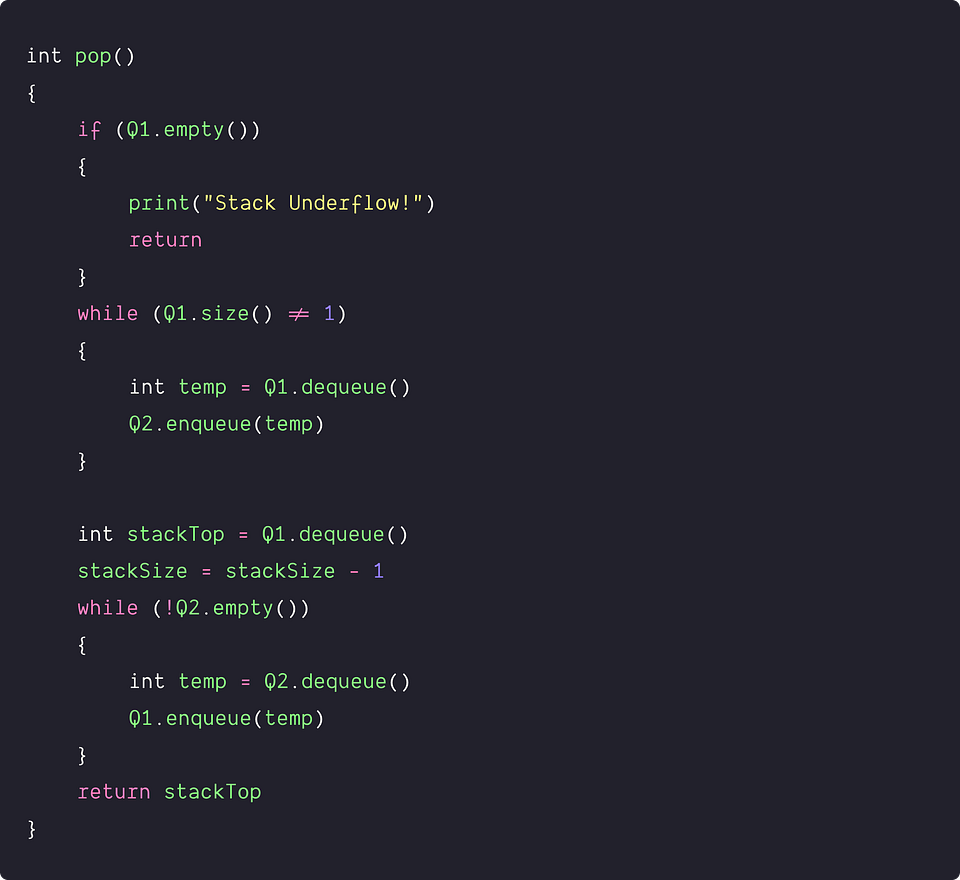Optimized implementation of the pop operation

The critical question is: in the 3rd step of the pop operation, do we really need to move all the elements from Q2 to Q1? If we observe closely, the answer is no: the Q1 will be empty at that stage, and elements in Q2 are in the same order as earlier in Q1. So rather than moving elements from Q2 to Q1, we just need to swap the reference of Q1 with Q2. Think!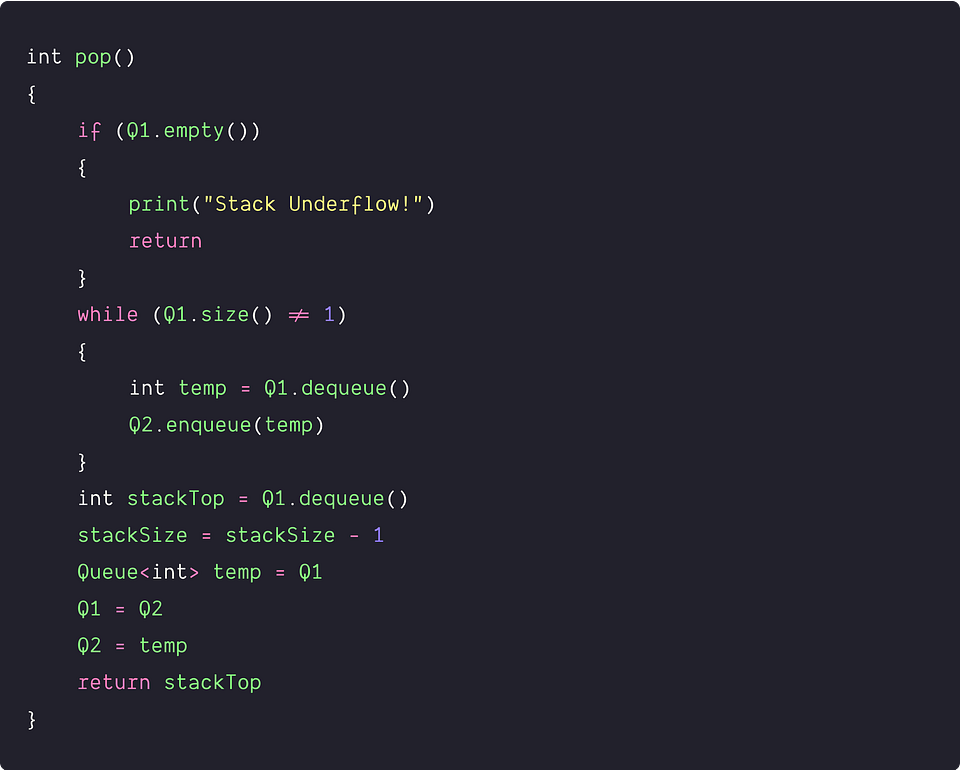• The algorithm dequeues n elements from Q1 and enqueues n − 1 element to Q2, where n is the size of the stack. So total number of queue operations = 2n − 1 and time complexity = O(n).
• We are using constant extra space. So space complexity = O(1)

Note: It's an exercise for you to design an effcient algorithm for the top() operation.

Class-based pseudocode implementation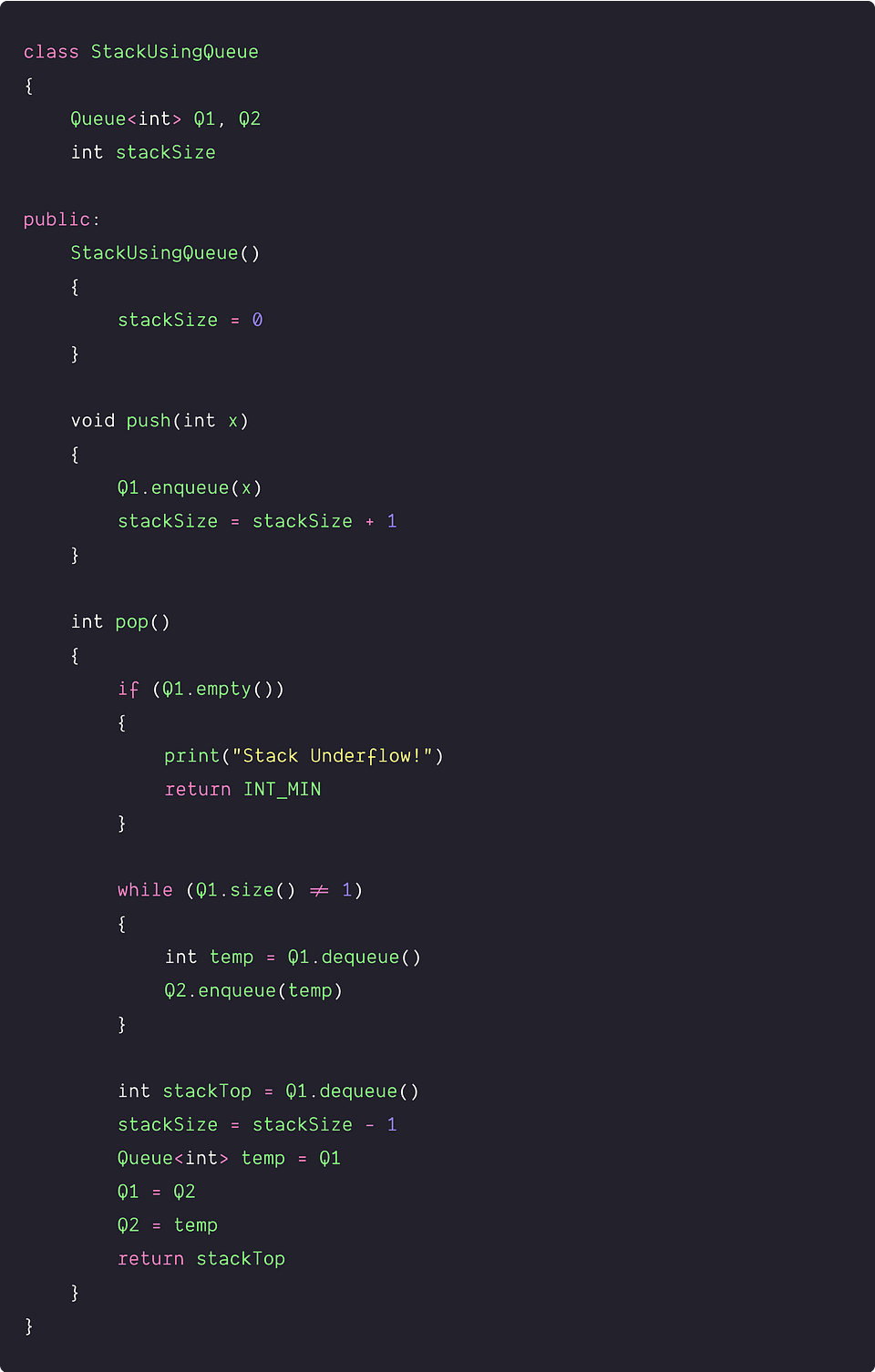### Using two queues: O(1) pop operation and O(n) push operation

Now the critical question is: can we think of another implementation similar to the above approach but in a reverse way i.e. O(1) pop and O(n) push operation? Let's think!

Implementation of the pop operation

Here the idea is to maintain the front element of the queue as the top element of the stack. So, for removing an element from the stack, we simply dequeue the front element from Q1.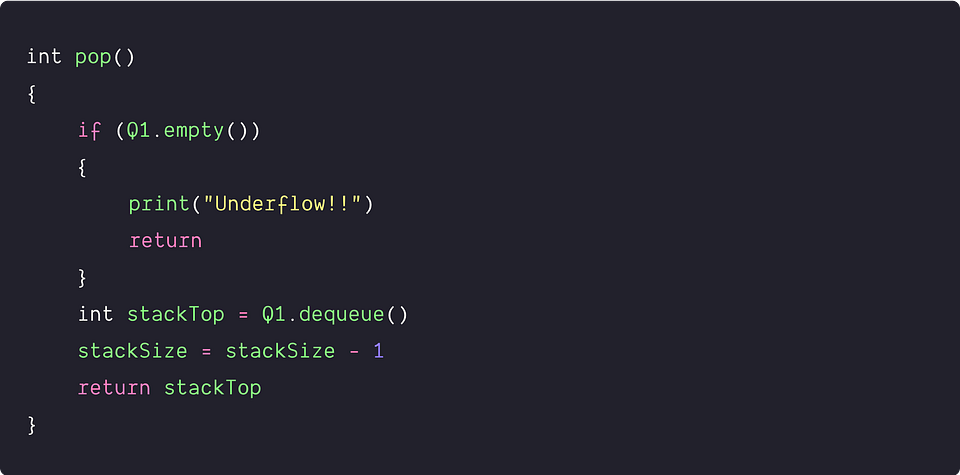• We are performing a constant number of operations i.e. only one dequeue operation. So time complexity = O(1)
• We are using constant extra space. So space complexity = O(1)

Implementation of the push operation

The new element is always inserted at the back of the queue. But for maintaining the stack order, we need to position the new element at the front of the queue. How do we do it? Let's think!

• We first move all elements from Q1 to Q2.
• Then we enqueue the new element x to the Q1.
• Now move all elements from Q2 to Q1. This ensures that the new element would be in the front of Q1. When we perform the next pop operation, we can easily access the new element from the front of the Q1.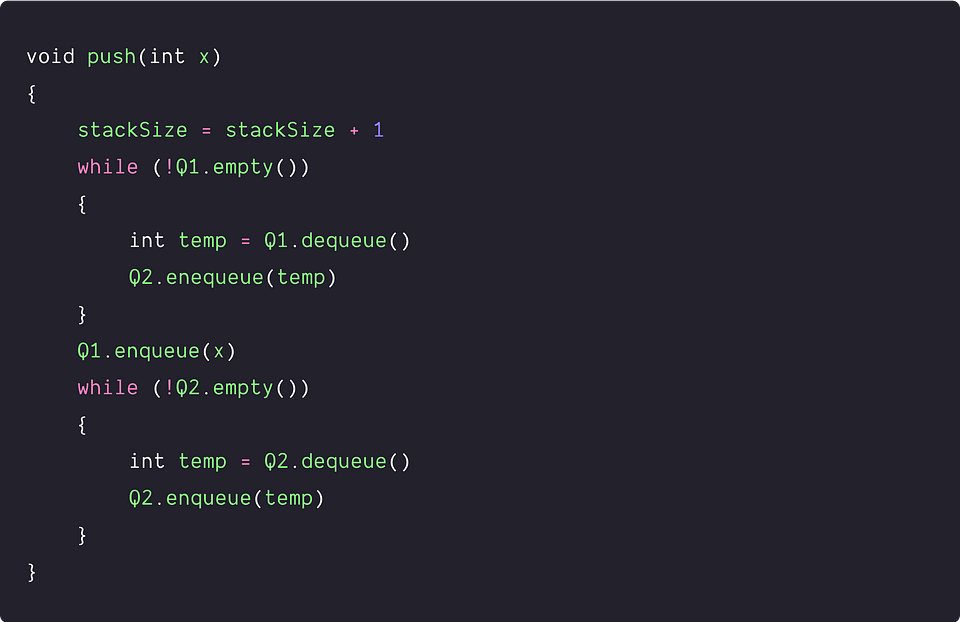Optimized implementation of the push operation

In the 3rd step of the push operation, there is no need to copy all the elements from Q2 to Q1. We have already discussed a similar idea for the pop operation in the above approach.

• We enqueue new element x to the empty queue Q2.
• Now we first move all elements from Q1 to Q2.
• After this, the new element will be present at the front of Q2 and Q1 is empty. So rather than copy elements from Q2 to Q1, we need to just swap the references of Q1 and Q2.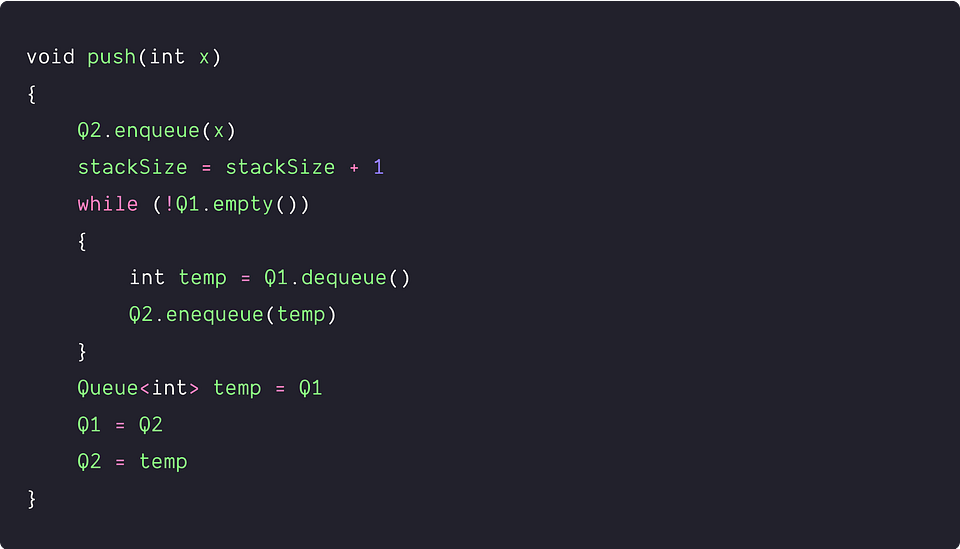• The algorithm removes n elements from Q1 and inserts n + 1 elements to Q2, where n is the size of the stack. So total number of queue operations = 2n + 1. Time complexity = O(n).
• Space complexity = O(1)

Note: It's an exercise for you to design an effcient algorithm for the top() operation.

Class-based pseudocode implementation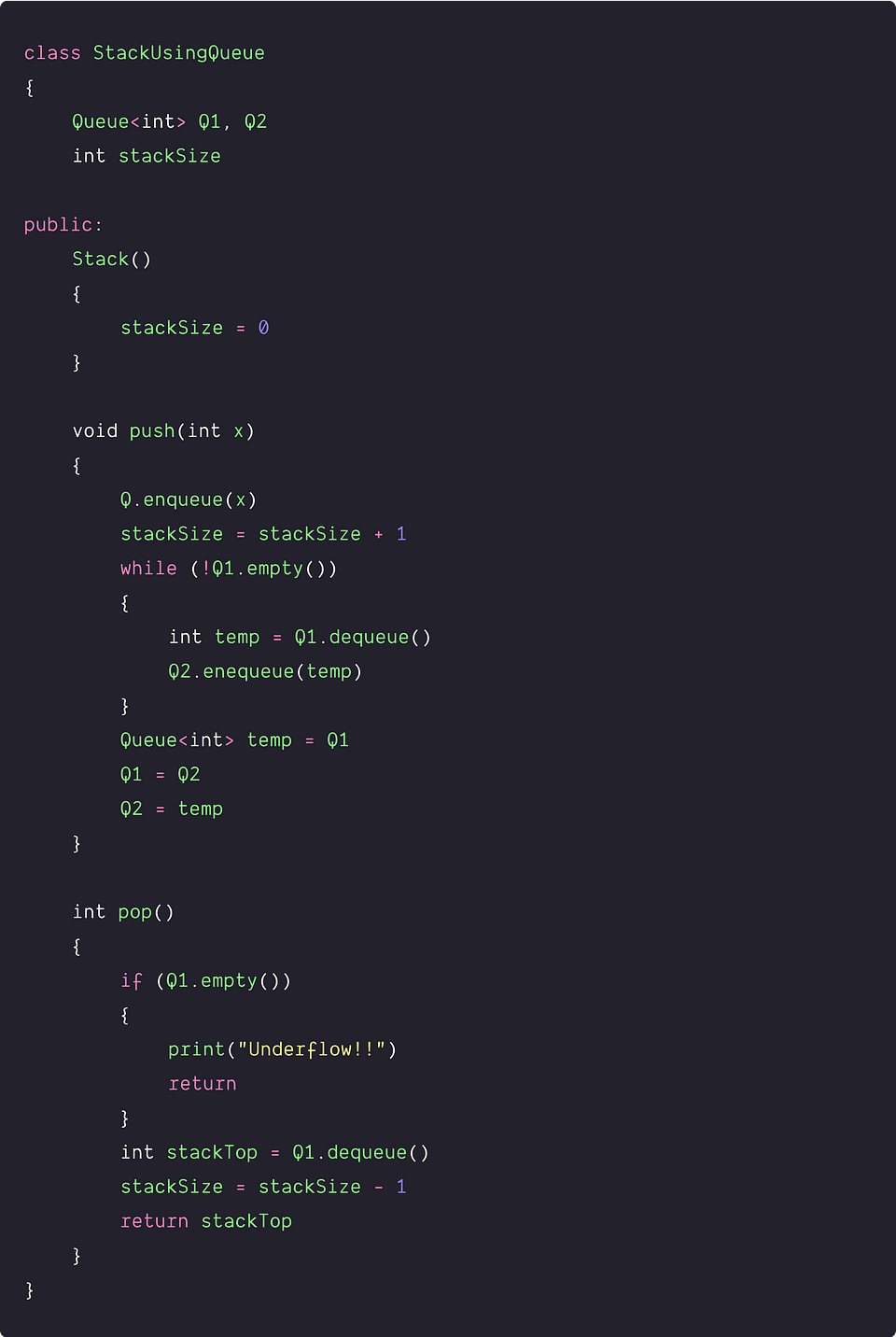### Using a single queue: O(1) pop operation and O(n) push operation

The above approaches use two queues. Now the critical question is: can we optimize the implementation using a single queue? Let's think!

Suppose for the pop operation; we always insert elements at the front of the queue to get access in constant time. Now for the push operation, if we insert an element in the queue, it will be stored at the back of the queue due to the queue properties. But we need the last inserted element to the front of the queue to remove it first in the next pop operation. One idea would be to: after pushing the new element, we need to reverse the order of the remaining queue elements. So we can use only one queue to implement the stack.

Implementation of the pop operation

For removing an element from the stack, we simply dequeue the front element from Q. This is similar to the previous approach.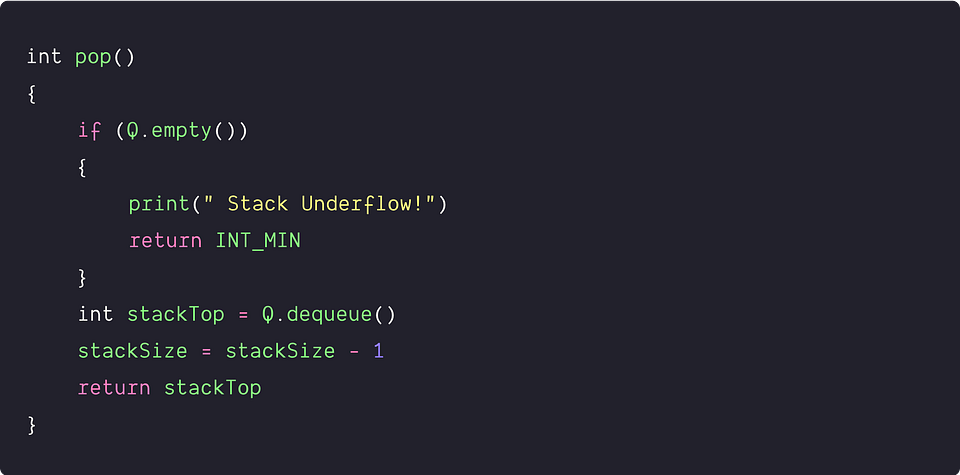• We are performing a constant number of operations i.e. only one dequeue operation. So time complexity = O(1)
• We are using constant extra space. So space complexity = O(1)

Implementation of the push operation

Here the idea is to first insert the new element x into the queue and then reverse the order of the queue elements. To reverse the order, we dequeue each element except the last element and enqueue it again to the queue. In other words, this process will run in a loop till there is a single element left in the queue.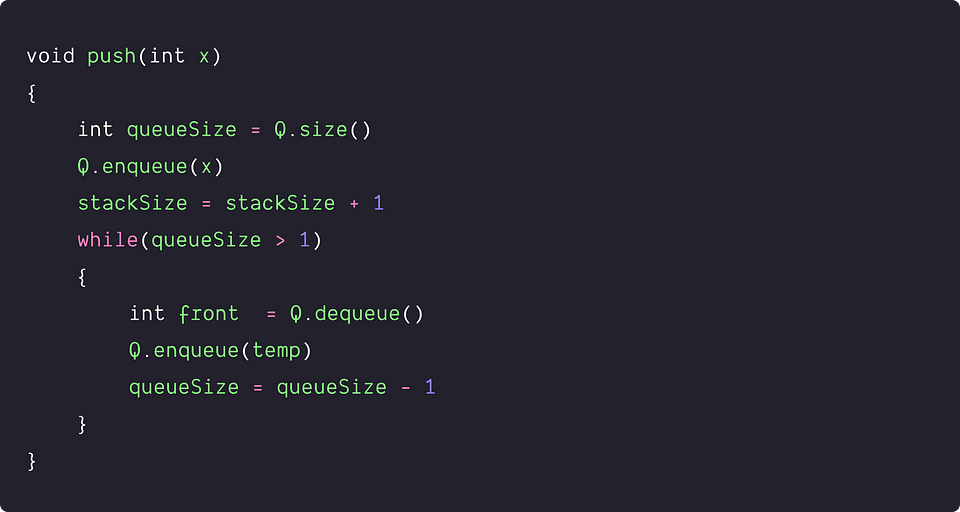• The algorithm removes n elements and inserts n+1 elements to Q, where n is the stack size. So total number of queue operations = 2n + 1. Time complexity = O(n).
• Space complexity = O(1).

Class-based pseudocode implementation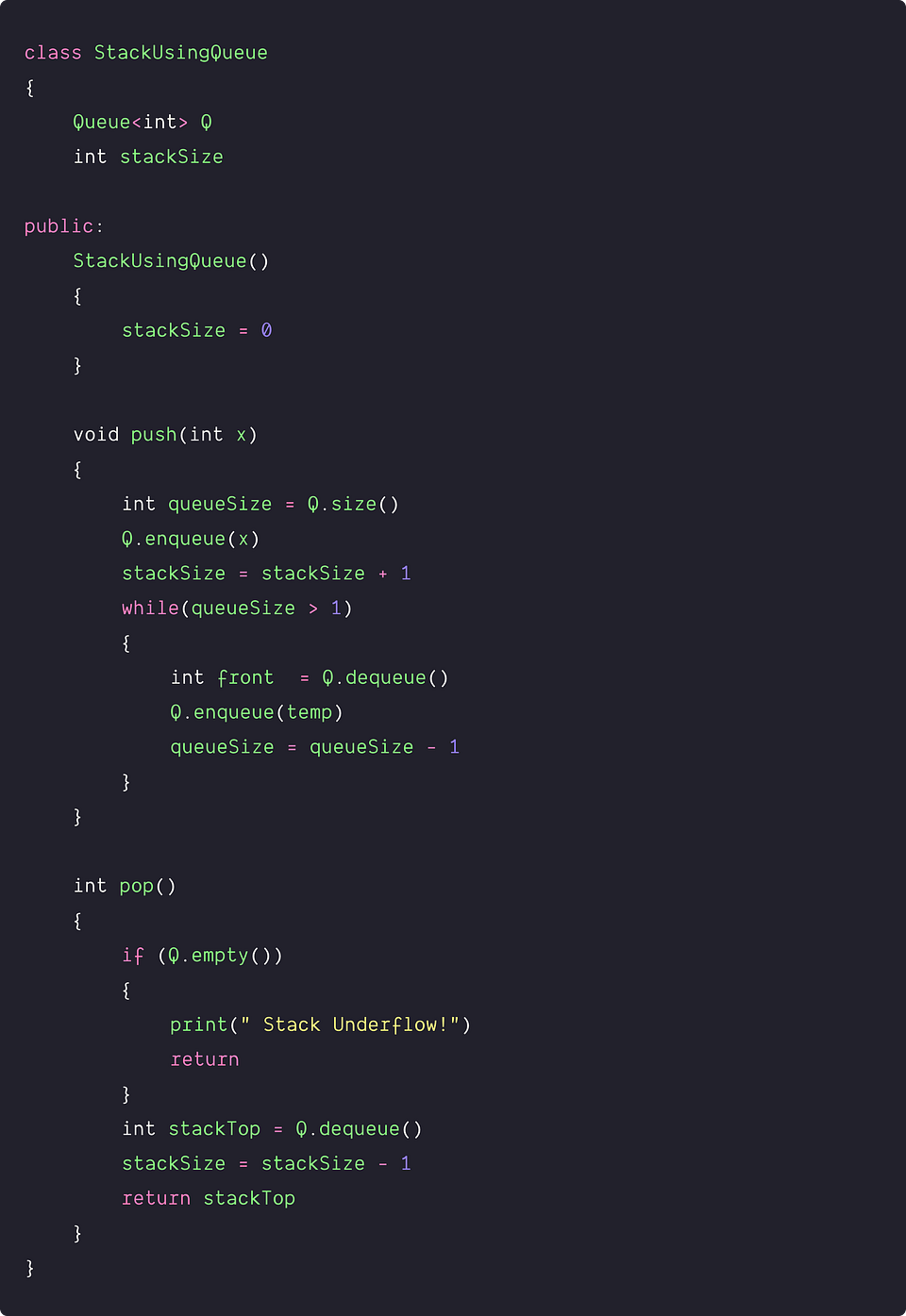### Critical ideas to think!

• Can we implement a stack where each operation is amortized O(1) time?
• How efficiency of the above approaches depends on the frequency of push and pop operations? Which approach should be followed if push operation is very high in comparison to the pop operation?
• Can we think of designing some other strategies to implement stack using queues?
• Based on the complexity of enqueue or dequeue operations, what type of queue implementation would be perfect for the above implementations? Array implementation or linked list implementation?
• How do we implement top() and isEmpty() operations in all the above approaches? Can we design a strategy to get the top element in O(1) time complexity?

### Suggested coding problems to practice

Enjoy learning, Enjoy Algorithms

Get well-designed application and interview centirc content on ds-algorithms, machine learning, system design and oops. Content will be delivered weekly.